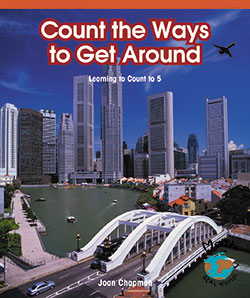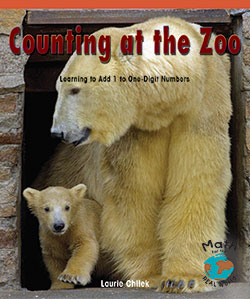# Math for the REAL World: Grades K – 1 (Levels A – D)

Math for the REAL World

Math for the REAL World is a program of leveled readers that integrate math, reading, and language. Each book addresses one of the NCTM focal points and connects the math to its real-life context. Each kit touches on the five strands of mathematics: • Number sense, properties, and operations • Measurement • Geometry and spatial sense • Data analysis, statistics, and probability • Algebra and functions Because Math for the REAL World leveled books connect computation and calculation to real-life concepts and applications, they address many important Science and Social Studies standards, as well as the academic language of Math and the content areas.

RL